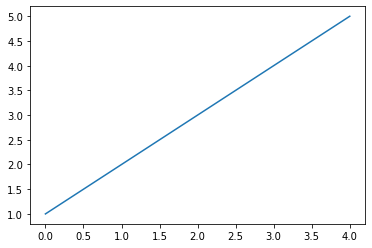# Scientific modules and IPython¶

Nikolay Koldunov

[email protected]

This is part of Python for Geosciences notes.

In :
%matplotlib inline
import matplotlib.pylab as plt


## Core scientific packages¶

When people say that they do their scientific computations in Python it's only half true. Python is a construction set, similar to MITgcm or other models. Without packages it's only a core, that although very powerful, does not seems to be able to do much by itself.

There is a set of packages, that almost every scientist would need:We are going to talk about all exept Sympy

## Installation¶

Installation instructions can be found in the README.md file of this repository. Better to use rendered version from GitHub.

## IPython¶

In order to be productive you need comfortable environment, and this is what IPython provides. It was started as enhanced python interactive shell, but with time become architecture for interactive computing.

## Jupyter notebook¶

Since the 0.12 release, IPython provides a new rich text web interface - IPython notebook. Here you can combine:

#### Code execution¶

In :
print('I love Python')

I love Python


#### Text (Markdown)¶

IPython website.

List:

Code:

print('hello world')

#### $\LaTeX$ equations¶

$$\int_0^\infty e^{-x^2} dx=\frac{\sqrt{\pi}}{2}$$$$F(x,y)=0 ~~\mbox{and}~~ \left| \begin{array}{ccc} F''_{xx} & F''_{xy} & F'_x \\ F''_{yx} & F''_{yy} & F'_y \\ F'_x & F'_y & 0 \end{array}\right| = 0$$

#### Plots¶

In :
x = [1,2,3,4,5]
plt.plot(x);#### Rich media¶

In :
from IPython.display import YouTubeVideo

Out:

## Run notebook¶

In order to start Jupyter notebook you have to type:

jupyter notebook

Web version can be accesed from the github repository.

## Main IPython features¶

### Getting help¶

You can use question mark in order to get help. To execute cell you have to press Shift+Enter

In :
?


Question mark after a function will open pager with documentation. Double question mark will show you source code of the function.

In :
plt.plot??


Press SHIFT+TAB after opening bracket in order to get help for the function (list of arguments, doc string).

In [ ]:
sum()


### Accessing the underlying operating system¶

You can access system functions by typing exclamation mark.

In :
!pwd

/Users/koldunovn/COURSE/python_for_geosciences


If you already have some netCDF file in the directory and ncdump is installed, you can for example look at its header.

In :
!ncdump -h test_netcdf.nc

netcdf test_netcdf {
dimensions:
TIME = 366 ;
LATITUDE = 94 ;
LONGITUDE = 192 ;
variables:
float TIME(TIME) ;
TIME:units = "hours since 1-1-1 00:00:0.0" ;
float LATITUDE(LATITUDE) ;
float LONGITUDE(LONGITUDE) ;
float New_air(TIME, LATITUDE, LONGITUDE) ;
New_air:missing_value = -9999.f ;
}


## Magic functions¶

The magic function system provides a series of functions which allow you to control the behavior of IPython itself, plus a lot of system-type features.

Let's create some set of numbers using range command:

In :
list(range(10))

Out:
[0, 1, 2, 3, 4, 5, 6, 7, 8, 9]

And find out how long does it take to run it with %timeit magic function:

In :
%timeit list(range(10))

396 ns ± 13 ns per loop (mean ± std. dev. of 7 runs, 1000000 loops each)


Print all interactive variables (similar to Matlab function):

In :
%whos

Variable       Type      Data/Info
----------------------------------
plt            module    <module 'matplotlib.pylab<...>ges/matplotlib/pylab.py'>
x              list      n=5


### Cell-oriented magic¶

Receive as argument both the current line where they are declared and the whole body of the cell.

In :
%%timeit
range(10)
range(100)

348 ns ± 5.51 ns per loop (mean ± std. dev. of 7 runs, 1000000 loops each)


Thre are several cell-oriented magic functions that allow you to run code in other languages:

In :
%%bash

echo "My shell is:" $SHELL  My shell is: /bin/bash  In : %%perl$variable = 1;
print "The variable has the value of \$variable\n";

The variable has the value of 1


You can write content of the cell to a file with %%writefile (or %%file for ipython < 1.0):

In :
%%writefile hello.py
#if you use ipython < 1.0, use %%file comand
#%%file
a = 'hello world!'
print(a)

Overwriting hello.py


And then run it:

In :
%run hello.py

hello world!

<Figure size 432x288 with 0 Axes>

The %run magic will run your python script and load all variables into your interactive namespace for further use.

In :
%whos

Variable       Type      Data/Info
----------------------------------

%magic